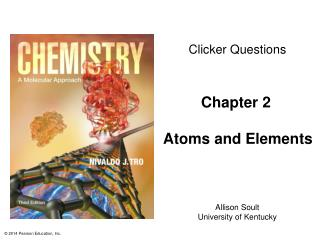DownloadDownload PresentationChapter 2 Atoms and Elements

# Chapter 2 Atoms and Elements

Télécharger la présentation## Chapter 2 Atoms and Elements

- - - - - - - - - - - - - - - - - - - - - - - - - - - E N D - - - - - - - - - - - - - - - - - - - - - - - - - - -
##### Presentation Transcript

1. Clicker Questions Chapter 2 Atoms and Elements Allison Soult University of Kentucky

2. Two samples of the same compound are compared:Sample 1: 24.22 g carbon and 32.00 g oxygen Sample 2: 36.22 g carbon and 48.00 g oxygenThis data shows which of the following? The law of definite proportions The law of multiple proportions The law of conservation of mass a and b only a, b, and c

3. What information about atomic structure was determined by Millikan’s oil drop experiment? • Presence of electrons • Presence of nucleus • Charge on an electron • Charge on a neutron • Charge on a proton

4. Which of the following statements about a carbon atom is correct according to the plum-pudding atom model? • Nothing can penetrate acarbon atom. • The carbon atom has apositive charge. • The positive charge isdistributed over thesphere of the atom. • The carbon atom has anegative charge.

5. Which scientist confirmed that most of an atom’s mass and all of its positive charge are contained in a small core called the nucleus? • John Dalton • Joseph Proust • J. J. Thomson • Ernest Rutherford • Robert Milliakn

6. Which of the following determines the identity ofan atom? • Number of protons • Number of electrons • Number of neutrons • Total number of protons and neutrons • Total number of protons and electrons

7. How many neutrons are in 1000 atoms of Cl-37? • 37 • 37,000 • 20 • 20,000 • Cannot be determined

8. How many protons are in an atom of 195Pt? • 273 • 195 • 117 • 78

9. Which of the following statements is true about ions? • An ion is the result of an atom gaining a neutron. • A positively charged ion results from the gain ofa proton. • A negatively charged ion results from the loss ofa proton. • The gain or loss of an electron by an atom willresult in an ion. • None of the above.

10. To form an ion, aluminum is most likely to • gain three electrons to become Al3–. • lose three electrons to become Al3+. • gain three protons to become Al3+. • lose three protons to become Al3–.

11. If a pure copper penny has 2.94 × 1022 atoms, what will be its mass? • 1.30 g • 0.323 g • 2.79 g • 3.10 g • 1.12 g

12. Which of the following elements is an alkaliearth metal? • Li • Fe • Ca • Ge • Pb

13. Which of the following elements will have properties most similar to tin, Sn (50)? • Lead, Pb (82) • Gallium, Ga (31) • Zinc, Zn (30) • Titanium, Ti (22) • Arsenic, As (33)

14. Boron has two naturally occurring isotopes: B-10 with an isotopic mass of 10.013 amu B-11 with an isotopic mass of 11.009 amuCalculate the percent abundance for each isotope if the average atomic mass of boron is 10.81 amu. 50.0% B-10, 50.0% B-11 25.0% B-10, 75.0% B-11 19.8% B-10, 80.2% B-11 26.4% B-10, 73.6% B-11 Not enough information to determine

15. The mass spectrum of gallium, Ga, is shown as follows. The atomic mass of Ga is 69.7 amu. Whichof the following statements is correct? • All Ga atoms weigh69.7 amu. • The atomic mass ofGa is the average of68.9 and 71.9. • The atomic mass of Gawill be closer to 69 than71 because there aremore atoms that weigh68.9 amu.

16. Which of the following is FALSE? • The mole can be used to specify Avogadro’s numberof anything. • Avogadro’s number, 6.022 × 1023, is an exactnumber. • The mole is equal to the number of atoms in exactly12 grams of carbon-12. • The value of an element’s molar mass in grams per mole is numerically equal to the element’s atomicmass in amu.

17. Which weighs more, a pea or a marble? • Pea • Marble

18. Which has more atoms, 10.0 g Mg or 10.0 g Ca? • Magnesium • Calcium • Both have the same number of atoms.

19. Calculate the mass in grams of one mole of footballs if one football has a mass of 0.43 kg. • 430 g • 1.4 g • 2.6 × 1026 g • 7.1 × 10–23 g • 1.4 × 1027 g

20. Which of the following has the largest mass? • 10.0 g Li • 10.0 moles of Li • 100 g Na • 10.0 moles of K • 100 g Rb